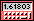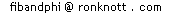# Hints to help you find the formulae for Fibonacci series problems

## Hints to start you off

Taking the first example above, the numbers don't seem to follow any immediately obvious pattern. There are several ways to proceed:

## Look at the differences

Here the differences are
```     Series:   1   2   4   7  12  20  ..
Differences 1   2   3   5   8    ..
```
and, if you've been following these pages closely, you should recognize the differences! However, this doesn't help us discover a pattern for the series itself.

If the differences are constant, that is, all the same, then the series is related to the square numbers

1, 4, 9, 16, 25, .. n2 .
If the differences of the differences are constant then the series is related to the cubes,
1, 8, 27, .. n3.

## Finding a Fibonacci-type relationship

For the series here, we can try adding the previous 2 terms and seeing if they are related in a simple way to the next term, in a similar manner to the Fibonacci numbers.
This is called finding a recurrence relation.
```  Series:  1   2   4   7  12  20 ..
1 + 2 = 3
2 + 4 = 6
4 + 7 =11
7 + 12=19
```
It looks like we have the sum of the previous two terms gives one less than the next term, or, in maths notation:
s(n-2)+s(n-1)=s(n)-1.
This we can check for a few more terms. If correct, it is an easier way to compute terms of the series without adding up lots of Fibonacci numbers, but it is not yet a formula for the series.

## Adding a constant to another series

If we take the differences of the Fibonacci numbers:
```  Fibonacci:  0  1  1  2  3  5  8 13 21 ..
Differences:  1  0  1  1  2  3  5 8 ..
```
we notice these are also the Fibonacci numbers. A moments thought should convince you that this must be true since we get the next Fibonacci number from the previous one by... adding in the Fibonacci number before that one.

Now we can see how our original series (1, 2, 4, 7, 12, 20,..) is made. Since its differences are the same as the Fibonacci series differences, we can add or subtract a constant to the Fibonacci series. If we add 1 to the each term of the series we get 2, 3, 5, 8, 13, 21.

The problem remaining is "Is it Fib(n)-1 or Fib(n+1)-1 or Fib(n-1)-1, or what?".

```
n:      1  2  3  4  5  6  ..
Series: 1  2  4  7 12 20  ..
Fib(n): 1  1  2  3  5  8 ..
so series is s(n) = Fib(n+2)-1
```
since each term of the series is one less than the Fibonacci number two further along.
##### now have a go yourself
..so try them for yourself. This is where Mathematics becomes more of an Art than a Science, since you are relying on your intuition to "spot" the pattern.

 0, 1, 1, 2, 3, 5, 8, 13, 21, 34, 55, 89, 144, 233, 377, 610, 987 ..More..Back to "Things to Do" problems© 1996-2006 Dr Ron KnottUpdated 1 October 1999